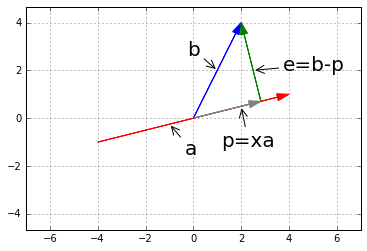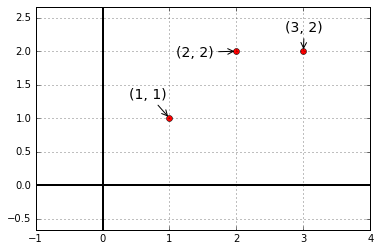# 第十五讲：子空间投影

$$\mathbb{R}^2$$空间讲起，有向量$$a, b$$，做$$b$$$$a$$上的投影$$p$$，如图：

%matplotlib inline
import matplotlib.pyplot as plt
import numpy as np
import pandas as pd

plt.style.use("seaborn-dark-palette")

fig = plt.figure()
plt.axis('equal')
plt.axis([-7, 7, -6, 6])
# plt.plot([48/17], [12/17], 'o')
# y=1/4x
# y=-4x+12
# x=48/17
# y=12/17
plt.annotate('b', xy=(1, 2), xytext=(-30, 15), textcoords='offset points', size=20, arrowprops=dict(arrowstyle="->"))
plt.annotate('a', xy=(-1, -0.25), xytext=(15, -30), textcoords='offset points', size=20, arrowprops=dict(arrowstyle="->"))
plt.annotate('e=b-p', xy=(2.5, 2), xytext=(30, 0), textcoords='offset points', size=20, arrowprops=dict(arrowstyle="->"))
plt.annotate('p=xa', xy=(2, 0.5), xytext=(-20, -40), textcoords='offset points', size=20, arrowprops=dict(arrowstyle="->"))
plt.grid()plt.close(fig)


$a^T(b-xa)=0 \\ \underline{xa^Ta=a^Tb} \\ \underline{x=\frac{a^Tb}{a^Ta}} \\ p=a\frac{a^Tb}{a^Ta}$

• $$\underline{P=P^T}$$，投影矩阵是一个对称矩阵。
• 如果对一个向量做两次投影，即$$PPb$$，则其结果仍然与$$Pb$$相同，也就是$$\underline{P^2=P}$$

$$Ax$$总是在$$A$$的列空间中，而$$b$$却不一定，这是问题所在，所以我们可以将$$b$$变为$$A$$的列空间中最接近的那个向量，即将无解的$$Ax=b$$变为求有解的$$A\hat{x}=p$$$$p$$$$b$$$$A$$的列空间中的投影，$$\hat{x}$$不再是那个不存在的$$x$$，而是最接近的解）。

• 第一个问题：$$\hat x=(A^TA)^{-1}A^Tb$$
• 第二个问题：$$p=A\hat x=\underline{A(A^TA)^{-1}A^T}b$$，回忆在$$\mathbb{R}^2$$中的情形，下划线部分就是原来的$$\frac{aa^T}{a^Ta}$$
• 第三个问题：易看出投影矩阵就是下划线部分$$P=A(A^TA)^{-1}A^T$$

## 最小二乘法

plt.style.use("seaborn-dark-palette")

fig = plt.figure()
plt.axis('equal')
plt.axis([-1, 4, -1, 3])
plt.axhline(y=0, c='black', lw='2')
plt.axvline(x=0, c='black', lw='2')

plt.plot(1, 1, 'o', c='r')
plt.plot(2, 2, 'o', c='r')
plt.plot(3, 2, 'o', c='r')

plt.annotate('(1, 1)', xy=(1, 1), xytext=(-40, 20), textcoords='offset points', size=14, arrowprops=dict(arrowstyle="->"))
plt.annotate('(2, 2)', xy=(2, 2), xytext=(-60, -5), textcoords='offset points', size=14, arrowprops=dict(arrowstyle="->"))
plt.annotate('(3, 2)', xy=(3, 2), xytext=(-18, 20), textcoords='offset points', size=14, arrowprops=dict(arrowstyle="->"))

plt.grid()plt.close(fig)